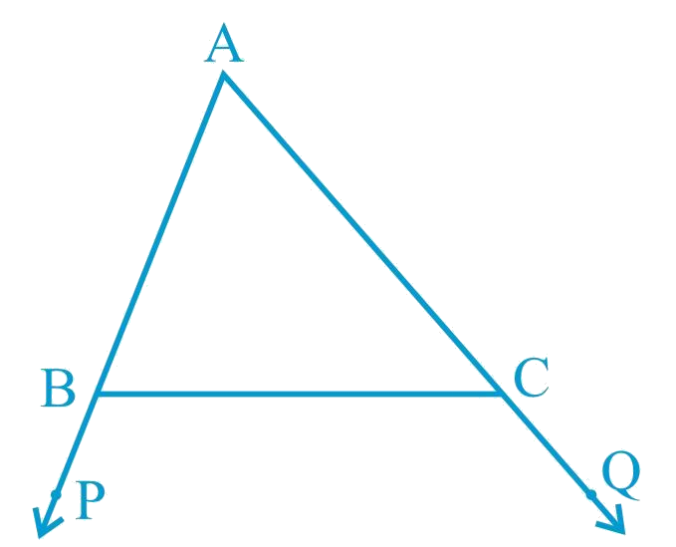# Ex.7.4 Q2 Triangles Solution - NCERT Maths Class 9

Go back to  'Ex.7.4'

## Question

In the given figure sides $$AB$$ and $$AC$$ of $$\Delta ABC$$ are extended to points $$P$$ and $$Q$$ respectively. Also, $$\angle PBC \lt \angle QCB$$. Show that $$AC \gt \angle AB$$.

Video Solution
Triangles
Ex 7.4 | Question 2

## Text Solution

What is Known?

$$\angle \text{PBC}<\angle \text{QCB}\text{.}$$

To prove:

$$\text{AC}>\text{AB}\text{.}$$

Reasoning:

By using linear pair, we can find inequality of interior angles and then we can use the fact that In any triangle, the side opposite to the larger (greater) angle is longer.Steps:

In the given figure,

\begin{align} &\angle ABC +\angle PBC=180^{\circ}\,\,\,\\&\text{( Linear pair)} \\\\& \angle ABC =180^{\circ} - \angle PBC\, \ldots (1) \\ \end{align}

Also,

\begin{align} &\angle ACB +\angle QCB=180^{\circ} \\ &\angle ACB =180^{\circ} - \angle QCB\, \ldots (2) \\ \end{align}

As $$\angle PBC \lt \angle QCB$$,

\begin{align} & 180^{\circ} - PBC \gt 180^{\circ} - \angle QCB\\ & \angle ABC \gt ACB\\&[\text{From Equations (1) and (2)}]\\\\ & AC \gt AB \\&\left (\begin{array}\ \text{side opposite to the}\\\text{ larger angle is larger.}\end{array} \right)\end{align}

Hence proved, $$AC \gt AB$$

Video Solution
Triangles
Ex 7.4 | Question 2

Learn from the best math teachers and top your exams

• Live one on one classroom and doubt clearing
• Practice worksheets in and after class for conceptual clarity
• Personalized curriculum to keep up with school